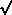1. The mean is most sensitive to extreme scores, because its computation considers the actual values of all the scores. It is the sum of all the scores, divided by the number of scores. One extreme score, added into that sum, can "throw off" the mean. The median, in contrast, is the score which cuts of 50% of the distribution, and therefore bearly is influenced by an extreme.

e.g., 3, 4, 5, 6, 32
The median here is 5, and is pretty representative of most of the scores. The score of 32 really throws off the mean, making it 10, rather than a value that seems representative of the other scores.

2. Simple effect: the effect of one independent variable at a single level of another independent variable
Main effect: the effect of one independent variable, ignoring the effect of the other independent variable(s). Another definition: the average of its simple effects
3. Two-way interaction: the extent to which the simple effects are different in direction and/or magnitude. Another definition: the systematic variability that remains once the main effects are taken into account

4. beta up power down
N up power up
s up power down

5. mean=(5+ 4+ 5+ 6+ 3+ 2+ 1)/7
Mode=5
Median = 4

6. The second set are mutually orthogonal, which can be proven by summing the cross-products of a pair of coefficients. -2(0)++1(-1)++1(+1) = 0

7. [310-[56(56)]/12]/12

8. lower limit is 10-2.369 (4/91)
upper limit is 10+2.369(4/91)

9. (80-90)/5
This computation would give you a negative z-score, so you would look up that z-score, to find the area under the curve that is beyond the z score. That would be the person's percentile rank.

10. There may be an interaction here, because the simple effect of mood for men is no change from happy to sad whereas the simple effect of mood for women is a +10 point change. If the simple effects differ in magnitude and/or direction, a 2way interaction is possible.

11.
SourceSSdfMSF
Total(307+146)-[(35+24)(35+24)]/88-1
Between[(35)(35)+(24)(24)]/4 -[(35+24)(35+24)]/8 2-1SSb/1MSb/MSw
WithinSSTotal-SSBetween8-2SSw/8-2